### Example 58.10 Combining Correlation Coefficients

This example combines sample correlation coefficients computed from a set of imputed data sets by using Fisher’s z transformation.

Fisher’s z transformation of the sample correlation r is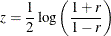The statistic z is approximately normally distributed with mean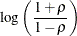and variance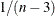, where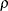is the population correlation coefficient and n is the number of observations.

The following statements use the CORR procedure to compute the correlation r and its associated Fisher’s z statistic between variables Oxygen and RunTime for each imputed data set. The ODS statement is used to save Fisher’s z statistic in an output data set.

proc corr data=outmi fisher(biasadj=no);
var Oxygen RunTime;
by _Imputation_;
ods output FisherPearsonCorr= outz;
run;


The following statements display the number of observations and Fisher’s z statistic for each imputed data set in Output 58.10.1:

proc print data=outz;
title 'Fisher''s Correlation Statistics';
var _Imputation_ NObs ZVal;
run;


Output 58.10.1: Output z Statistics

 Fisher's Correlation Statistics

Obs _Imputation_ NObs ZVal
1 1 31 -1.27869
2 2 31 -1.30715
3 3 31 -1.27922
4 4 31 -1.39243
5 5 31 -1.40146

The following statements generate the standard error associated with the z statistic,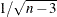:

data outz;
set outz;
StdZ= 1. / sqrt(NObs-3);
run;


The following statements use the MIANALYZE procedure to generate a combined parameter estimateand its variance, as shown in Output 58.10.2. The ODS statement is used to save the parameter estimates in an output data set.

proc mianalyze data=outz;
ods output ParameterEstimates=parms;
modeleffects ZVal;
stderr StdZ;
run;


Output 58.10.2: Combining Fisher’s z Statistics

The MIANALYZE Procedure

Parameter Estimates
Parameter Estimate Std Error 95% Confidence Limits DF Minimum Maximum Theta0 t for H0:
Parameter=Theta0
Pr > |t|
ZVal -1.331787 0.200327 -1.72587 -0.93771 330.23 -1.401459 -1.278686 0 -6.65 <.0001

In addition to the estimate for z, PROC MIANALYZE also generates 95% confidence limits for z,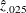and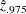. The following statements print the estimate and 95% confidence limits for z in Output 58.10.3:

proc print data=parms;
title 'Parameter Estimates with 95% Confidence Limits';
var Estimate LCLMean UCLMean;
run;


Output 58.10.3: Parameter Estimates with 95% Confidence Limits

 Parameter Estimates with 95% Confidence Limits

Obs Estimate LCLMean UCLMean
1 -1.331787 -1.72587 -0.93771

An estimate of the correlation coefficient with its corresponding 95% confidence limits is then generated from the following inverse transformation as described in the section Correlation Coefficients: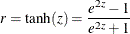for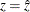,, and.

The following statements generate and display an estimate of the correlation coefficient and its 95% confidence limits, as shown in Output 58.10.4:

data corr_ci;
set parms;
r=       tanh( Estimate);
r_lower= tanh( LCLMean);
r_upper= tanh( UCLMean);
run;
proc print data=corr_ci;
title 'Estimated Correlation Coefficient'
' with 95% Confidence Limits';
var r r_lower r_upper;
run;


Output 58.10.4: Estimated Correlation Coefficient

 Estimated Correlation Coefficient with 95% Confidence Limits

Obs r r_lower r_upper
1 -0.86969 -0.93857 -0.73417